# The QLIM Procedure

### ENDOGENOUS Statement

Subsections:
• ENDOGENOUS variables ~ options ;

The ENDOGENOUS statement specifies the type of dependent variables that appear on the left-hand side of the equation. Endogenous variables listed refer to the dependent variables that appear on the left-hand side of the equation.

#### Discrete Variable Options

DISCRETE <(discrete-options )>

specifies that the endogenous variables in this statement are discrete. Valid discrete-options are as follows:

ORDER=DATA | FORMATTED | FREQ | INTERNAL

specifies the sorting order for the levels of the discrete variables specified in the ENDOGENOUS statement. This ordering determines which parameters in the model correspond to each level in the data. The following table shows how PROC QLIM interprets values of the ORDER= option.

 Value of ORDER= Levels Sorted By DATA Order of appearance in the input data set FORMATTED Formatted value FREQ Descending frequency count; levels with the most observations come first in the order INTERNAL Unformatted value

By default, ORDER=FORMATTED. For the values FORMATTED and INTERNAL, the sort order is machine dependent. For more information about sorting order, see the chapter on the SORT procedure in the Base SAS Procedures Guide.

DISTRIBUTION=NORMAL |LOGISTIC
DIST=NORMAL |LOGISTIC
D=NORMAL |LOGISTIC

specifies the cumulative distribution function used to model the response probabilities. DISTRIBUTION=NORMAL specifies the normal distribution for the probit model. DISTRIBUTION=LOGISTIC specifies the logistic distribution for the logit model.

By default, DISTRIBUTION=NORMAL.

If a multivariate model is specified, logistic distribution is not allowed. Only normal distribution is supported.

#### Censored Variable Options

CENSORED (censored-options )

specifies that the endogenous variables in this statement be censored. Valid censored-options are as follows:

LB=value or variable
LOWERBOUND=value or variable

specifies the lower bound of the censored variables. If value is missing or the value in variable is missing, no lower bound is set. By default, no lower bound is set.

UB=value or variable
UPPERBOUND=value or variable

specifies the upper bound of the censored variables. If value is missing or the value in variable is missing, no upper bound is set. By default, no upper bound is set.

#### Truncated Variable Options

TRUNCATED (truncated-options )

specifies that the endogenous variables in this statement be truncated. Valid truncated-options are as follows:

LB=value or variable
LOWERBOUND=value or variable

specifies the lower bound of the truncated variables. If value is missing or the value in variable is missing, no lower bound is set. By default, no lower bound is set.

UB=value or variable
UPPERBOUND=value or variable

specifies the upper bound of the truncated variables. If value is missing or the value in variable is missing, no upper bound is set. By default, no upper bound is set.

#### Stochastic Frontier Variable Options

FRONTIER <(frontier-options )>

specifies that the endogenous variable in this statement follow a production or cost frontier. Valid frontier-options are as follows:

TYPE=HALF |EXPONENTIAL |TRUNCATED

specifies the model type:

HALF

specifies a half-normal model.

EXPONENTIAL

specifies an exponential model.

TRUNCATED

specifies a truncated normal model.

PRODUCTION

specifies that the model estimated be a production function.

COST

specifies that the model estimated be a cost function.

If neither PRODUCTION nor COST option is specified, production function is estimated by default.

#### Selection Options

SELECT (select-option )

specifies selection criteria for sample selection model. The BAYES statement does not support the SELECT option. The select-option specifies the condition for the endogenous variable to be selected. It is written as a variable name, followed by an equality operator (=) or an inequality operator (<, >, <=, >=), followed by a number:

• variable operator number

The variable is the endogenous variable that the selection is based on. The operator can be =, <, >, <= , or >=. Multiple select-options can be combined with the logic operators: AND, OR. The following example illustrates the use of the SELECT option:

```   endogenous y1 ~ select(z=0);
endogenous y2 ~ select(z=1 or z=2);
```

The SELECT option can be used together with the DISCRETE, CENSORED, or TRUNCATED option. For example:

```   endogenous y1 ~ select(z=0) discrete;
endogenous y2 ~ select(z=1) censored (lb=0);
endogenous y3 ~ select(z=1 or z=2) truncated (ub=10);
```

For more information about selection models with censoring or truncation, see the section Selection Models.

#### Endogeneity and Overidentification Test Options

ENDOTEST (regressors)

requests the test of endogeneity for a list of regressors in the model. More specifically, this option tests the null hypothesis that the specified regressors are exogenous. Each of these regressors must also have a model of its own. The former model is considered the structural model, and the latter models are considered reduced form models.

The following example illustrates the use of the ENDOTEST option by testing whether the regressors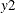and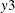are endogenous in the model for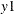:

```   proc qlim;
model y1 = y2 y3 x1;
model y2 = x1 x2 x3 x4 x5;
model y3 = x1 x2 x3 x4 x5;
endogenous y1 ~ endotest(y2 y3);
run;
```

The ENDOTEST option is not available when you specify the SELECT or FRONTIER option. You can specify the ENDOTEST option only once for each ENDOGENOUS statement.

For more information about the test for endogeneity, see the section Test for Endogeneity.

OVERID (variables)

requests the overidentification test for a list of variables. These variables are the overidentifying instrumental variables that you provide from the reduced form models. For more information, see the section Overidentification Test.

The following example illustrates the use of the OVERID option:

```   proc qlim;
model y1 = y2 y3 x1;
model y2 = x1 x2 x3 x4 x5;
model y3 = x1 x2 x3 x4 x5;
endogenous y1 ~ overid(y2.x4 y3.x5);
run;
```

The regressorsandin the model forare the endogenous variables. Therefore, each of these variables has its own models, which are considered reduced form models. The overidentifying instrumental variables are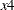and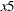. If you specify the OVERID option as

```      endogenous y1 ~ overid(y2.x4 y2.x5);
```

then you consider only the regressorto be endogenous, and the model foris ignored during the testing process.

The OVERID option is not available when you specify the SELECT or FRONTIER option. You can specify the OVERID option only once for each ENDOGENOUS statement.